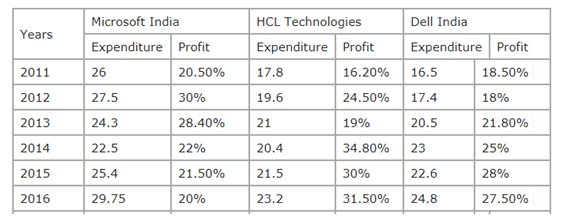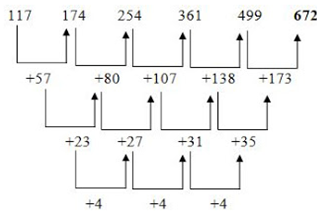# “20-20” Quantitative Aptitude | Crack SBI Clerk 2018 Day-29

Dear Friends, SBI Clerk 2018 Notification has been released we hope you all have started your preparation. Here we have started New Series of Practice Materials specially for SBI Clerk 2018. Aspirants those who are preparing for the exams can use this “20-20” Quantitative Aptitude Questions.

## Daily Practice Test Schedule | Good Luck

 Topic Daily Publishing Time Daily News Papers & Editorials 8.00 AM Current Affairs Quiz 9.00 AM Logical Reasoning 10.00 AM Quantitative Aptitude “20-20” 11.00 AM Vocabulary (Based on The Hindu) 12.00 PM Static GK Quiz 1.00 PM English Language “20-20” 2.00 PM Banking Awareness Quiz 3.00 PM Reasoning Puzzles & Seating 4.00 PM Daily Current Affairs Updates 5.00 PM Data Interpretation / Application Sums (Topic Wise) 6.00 PM Reasoning Ability “20-20” 7.00 PM English Language (New Pattern Questions) 8.00 PM General / Financial Awareness Quiz 9.00 PM

[WpProQuiz 1343]

Click “Start Quiz” to attend these Questions and view Explanation

### Click Here for SBI Clerk Prelims and Mains 2018 – Full Length Mock Test

Direction (Q. 1-5): What will come in place of the question mark (?) in the following number series?

1. 23, ? , 53, 163, 657, 3291
1. 32
2. 52
3. 26
4. 25
5. 37
1. 187, 17, 119, 23.8, ? , 35.7
1. 61.2
2. 71.4
3. 54.3
4. 68.6
5. None of these
1. 117, 174, 254, 361, 499, ?
1. 652
2. 784
3. 672
4. 562
5. 799
1. 7, 4.5, 7.5, 16.25, 39.5, ?, 334.25
1. 80.75
2. 120.25
3. 120.75
4. 107.75
5. 108
1. 42, 45, 57, 87, 143, ? , 460
1. 233
2. 243
3. 232
4. 195
5. 283

Directions (Q. 6-10): What will come in place of question mark (?) in the following questions?

6). 3/8 of 4/9 of 1575 + (√?) – 32% of 786 = 66.98

1. √56
2. 56
3. (56)2
4. 36
5. (36)2
1. 3√97336 +√6889 ÷ x– 3/4 of 64 – 52 = 43
1. 76/95
2. 53/79
3. 57/79
4. 83/97
5. None of these

8). √33124 × √2601− 832 = ?2 +372

1. 37
2. 32
3. 28
4. 29
5. None

9). 572 ÷ 26 × 12 – 200 = (2)?

1. 5
2. 6
3. 7
4. 8
5. None of these

10). 4  51/13 × 8  3/37 × 1  7/12 × [(16)2 + 3 ] = 23 × ?

1. 1141.584
2. 1151.584
3. 11415.84
4. 11515.84
5. None of these
1. A, B and C can finish a work in 10 days. A alone can do it in 24 days while B can do it in 30 days. A and B work together for 12 days and leave the rest of the work and it to be completed by C. How many days will C take to do the rest of the work?
1. 4
2. 6
3. 8
4. 5
5. 7
1. A certain number of men can finish a piece of work in 100 days. If, however, there was 10 men less, it would take 10 days more for the work to be finished. How many men were there originally?
1. 100
2. 82
3. 75
4. 110
5. 234
1. A shopkeeper sold sarees at Rs 266 each after giving 5% discount on labelled price. Had he not given the discount, he would have earned a profit of 12% on the cost price. What was the cost price of each saree?
1. 300
2. 345
3. 240
4. 250
5. none of these
1. An amount of Rs 2430 is divided between A,B and C such that if their shares be reduced by Rs 5, Rs 10 and Rs 15 respectively, the remainders shall be in the ratio of 3:4:5. Then find the share of B
1. 880
2. 780
3. 800
4. 1000
5. none of these.
1. 5 years ago the age of a man was 3 years more than 4 times his son’s age. After 3 years the man will be 6 years less than thrice the age of his son. After how many years will the sum of their ages be 80 years?
1. 32
2. 16
3. 24
4. 45
5. 32

Directions (Q. 16-20): The following table shows the expenditure (in crore) of three companies HCL Technologies, Dell India and Microsoft India and the percentage profit of these companies in different years.1. The income of Dell India in the year 2014 is approximately what per cent of the expenditure of HCL Technologies in the year 2013?
1. 147%
2. 125%
3. 131%
4. 140%
5. 137%
1. The expenditure of HCL Technologies in the year 2011 and 2016 together is approximately what percent of the expenditure of Microsoft India in the year 2012 and 2014 together?
1. 77%
2. 80%
3. 85%
4. 82%
5. 88%
1. The percentage profit of Microsoft India in the year 2013 is approximately what per cent more or less than the percentage profit of HCL Technologies in the year 2011?
1. 75%
2. 70%
3. 79%
4. 83%
5. 89%
1. What is the income of Microsoft India in the year 2015?
1. 33.251 crore
2. 28.652 crore
3. 30.861 crore
4. 24.543 crore
5. 35.786 crore
1. What is the difference between the profits of HCL Technologies and Dell India in the year 2016?
1. 46.2 lakh
2. 48.8 lakh
3. 50.9 lakh
4. 44.7 lakh
5. 52.2 lakh

Direction (Q. 1-5):

The pattern of the number series is ×1+2, ×2+3, ×3 +4, × 4+5, ×5+6

23×1+2 = 25

25×2+3 = 53

53×3 +4 = 163

163×4+5 = 657

821×5+6 = 3291

The pattern of the number series is ÷ 11, ×7, ÷ 5, ×3, ÷ 2

187÷ 11= 17

17× 7 = 119

119÷ 5 = 23.8

23.8× 3 =71.4

71.4÷ 2 = 35.7The pattern of the number series is ×0.5+1, ×1+3, ×1.5+5, ×2+7, ×2.5+9

7× 0.5 +1 = 4.5

4.5×1 +3 = 7.5

7.5×1.5 +5 = 16.25

16.25×2 +7 = 39.50

39.50×2.5+9 = 107.75

107.75×3 +11 = 334.25

The difference of difference of difference is, 9,8,7,6…

Directions (Q. 6-10):

3/8 × 4/9 × 1575 + (√?) – 32% of 786 = 66.98

1/2 × 525 + (√?) – (32/100) × 786 = 66.98

262.5 + (√?) – 251.52 = 66.98

(√?) = 66.98 + 251.52 -262.5

(√?)= 56

? = (56)2

3√97336 +√6889 ÷ x – 3/4 of 64 – 52 = 43

46 + 83/x – 48 – 52 = 43

83/x = 43+100 – 46

83/x = 97

X= 83/97

√33124 × √2601− 832 = ?2 + 372

182 x 51 – 6889 = ?2 + 1369

2393=?2 + 1369

(?)2 = 2393 −1369 =1024

? = √1024 = 32

572 ÷ 26 × 12 – 200 = (2)?

264-200 = 2?

64 =2?

2? = 26

X=6

23 x = 103/ 13 x 299/ 37 x 19/12 x 259

x=1141.584

Total units of work= 120

A’s one day work= 5 units

B’s one day work= 4 units

A + B+C one day work= 12 units

C’s one day work= 12-(5+4) = 3 units

Remaining work = 120-(9*12) = 12 units

Time required by C to complete the work= 12/3= 4 days

Let x men can finish the work in 100 days

x – 10 men can finish the work in 110 days

By M.D.H rule

x*100= 110(x-10)

100x= 110x- 1100

x= 110 men

Labelled price of a saree= 266/95*100= Rs 280

Cost price of the saree= 280/112*100= Rs 250

Remainder amount after deducting their share= 2430- (5+10+15) = Rs 2400

Thus, B’s share= 2400*4/12= Rs 800.

Let the present age of the man and his son be x and y respectively

So,

x – 5= 4(y-5) +3

x – 4y= -12….. (i)

Again

X+3 = 3(y+3) -6

x – 3y= 0…….(ii)

By solving, we get

X= 36,y=12

Sum of their ages = 48

Hence after 16 years the sum of their ages will be 80 years.

Directions (Q. 16-20):

Income of Dell India in 2014

= 23 + 23 × 25/100

= 28.75 crore

Expenditure of HCL Technologies in 2013 = 21 crore

Required % = 28.75/21 × 100

= 136.9 %

= 137%

Expenditure of HCL Technologies

= 17.8 + 23.2 = 41 crore

Expenditure of Microsoft India

= 27.5 + 22.5 = 50 crore

Required% = (41/50) × 100 = 82%

Percentage profit of Microsoft India in 2009 = 28.4%

Percentage profit of HCL Technologies in 2011 = 16.2%

Required % = (28.4 – 16.2)/16.2 × 100

= 12.2 × 100/16.2

= 75.3%

= 75%

Income of Microsoft India in 2015

= 25.4 + 25.4 × 21.5/100

= 25.4 + 5.461

= 30.861 crore

Profit of HCL Technologies in 2016

= 23.2 × 31.5 /100

= 7.308

Profit of Dell India in 2016

= 24.8 × 27.5/100

= 6.82

Difference = 7.308 – 6.820

= 0.488 crore

= 48.8 lakh